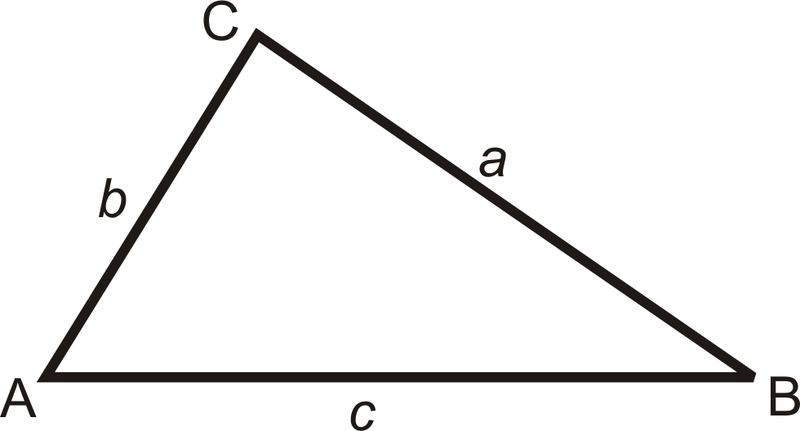# 4.36: Distance and Triangle Classification Using the Pythagorean Theorem

•• Contributed by CK12
• CK12

Find missing sides to calculate the area.

## Applications of the Pythagorean Theorem

### Find the Height of an Isosceles Triangle

One way to use The Pythagorean Theorem is to find the height of an isosceles triangle (see Example 1).Figure $$\PageIndex{1}$$

#### Prove the Distance Formula

Another application of the Pythagorean Theorem is the Distance Formula. We will prove it here.Figure $$\PageIndex{2}$$

Let’s start with point $$A(x_1,y_1)$$ and point $$B(x_2, y_2)$$. We will call the distance between $$A$$ and $$B$$, $$d$$.

Draw the vertical and horizontal lengths to make a right triangle.Figure $$\PageIndex{3}$$

Now that we have a right triangle, we can use the Pythagorean Theorem to find the hypotenuse, $$d$$.

\begin{align*} d^2=(x_1−x_2)^2+(y_1−y_2)^2 \\ d=\sqrt{(x_1−x_2)^2+(y_1−y_2)^2} \end{align*}

Distance Formula: The distance between $$A(x_1, y_1)$$ and $$B(x_2, y_2)$$ is $$d=\sqrt{(x_1−x_2)^2+(y_1−y_2)^2}$$.

#### Classify a Triangle as Acute, Right, or Obtuse

We can extend the converse of the Pythagorean Theorem to determine if a triangle is an obtuse or acute triangle.

Acute Triangles: If the sum of the squares of the two shorter sides in a right triangle is greater than the square of the longest side, then the triangle is acute.Figure $$\PageIndex{4}$$

For $$b<c$$ and $$a<c$$, if $$a^2+b^2>c^2$$, then the triangle is acute.

Obtuse Triangles: If the sum of the squares of the two shorter sides in a right triangle is less than the square of the longest side, then the triangle is obtuse.Figure $$\PageIndex{5}$$

For $$b<c$$ and $$a<c$$, if $$a^2+b^2<c^2$$, then the triangle is obtuse.

What if you were given an equilateral triangle in which all the sides measured 4 inches? How could you use the Pythagorean Theorem to find the triangle's altitude?

Example $$\PageIndex{1}$$

What is the height of the isosceles triangle?Figure $$\PageIndex{6}$$

Solution

Draw the altitude from the vertex between the congruent sides, which will bisect the base.Figure $$\PageIndex{7}$$

\begin{align*} 7^2+h^2&=9^2 \\ 49+h^2&=81 \\ h^2&=32 \\ h&=\sqrt{32}=\sqrt{16\cdot 2}=4\sqrt{2}\end{align*}

Example $$\PageIndex{2}$$

Find the distance between $$(1, 5)$$ and $$(5, 2)$$.

Solution

Make $$A(1,5)$$ and $$B(5,2)$$. Plug into the distance formula.

\begin{align*} d &=\sqrt{(1−5)^2+(5−2)^2} \\ &=\sqrt{(−4)^2+(3)^2} \\ &=\sqrt{16+9}=\sqrt{25}=5\end{align*}

Just like the lengths of the sides of a triangle, distances are always positive.

Example $$\PageIndex{3}$$

Graph $$A(−4,1)$$, $$B(3,8)$$, and $$C(9,6)$$. Determine if $$\Delta{ABC}$$ is acute, obtuse, or right.Figure $$\PageIndex{8}$$

Solution

Use the distance formula to find the length of each side.

\begin{align*} AB&=\sqrt{(−4−3)^2+(1−8)^2}=\sqrt{49+49}=\sqrt{98} \\ BC &=\sqrt{(3−9)^2+(8−6)^2}=\sqrt{36+4}=\sqrt{40} \\ AC &=\sqrt{(−4−9)^2+(1−6)^2}=\sqrt{169+25}=\sqrt{194}\end{align*}

Plug these lengths into the Pythagorean Theorem.

\begin{align*} \sqrt{98})^2+(\sqrt{40})^2 &? (\sqrt{194})^2 \\ 98+40 &? 194 \\ 138 &< 194\end{align*}

$$\Delta{ABC}$$ is an obtuse triangle.

For Examples 4 and 5, determine if the triangles are acute, right or obtuse.

Example $$\PageIndex{4}$$

Set the longest side to c.Figure $$\PageIndex{9}$$

Solution

\begin{align*} 15^2+14^2 &? \: 21^2 \\ 225+196 &? \: 441 \\ 421 &< 441\end{align*}

The triangle is obtuse.

Example $$\PageIndex{5}$$

Set the longest side to $$c$$.

Solution

A triangle with side lengths 5, 12, 13.

$$5^2+12^2=13^2$$ so this triangle is right.

## Review

Find the height of each isosceles triangle below. Simplify all radicals.

1.Figure $$\PageIndex{10}$$
2.Figure $$\PageIndex{11}$$
3.Figure $$\PageIndex{12}$$

Find the length between each pair of points.

1. $$(-1, 6)$$ and $$(7, 2)$$
2. $$(10, -3)$$ and $$(-12, -6)$$
3. $$(1, 3)$$ and $$(-8, 16)$$
4. What are the length and width of a 42” HDTV? Round your answer to the nearest tenth.
5. Standard definition TVs have a length and width ratio of 4:3. What are the length and width of a 42” Standard definition TV? Round your answer to the nearest tenth.

Determine whether the following triangles are acute, right or obtuse.

1. 7, 8, 9
2. 14, 48, 50
3. 5, 12, 15
4. 13, 84, 85
5. 20, 20, 24
6. 35, 40, 51
7. 39, 80, 89
8. 20, 21, 38
9. 48, 55, 76

Graph each set of points and determine whether \Delta{ABC} is acute, right, or obtuse, using the distance formula.

1. $$A(3,−5), B(−5,−8), C(−2,7)$$
2. $$A(5,3), B(2,−7), C(−1,5)$$
3. $$A(1,6), B(5,2), C(−2,3)$$
4. $$A(−6,1), B(−4,−5), C(5,−2)$$

## Vocabulary

Term Definition
acute triangle A triangle where all angles are less than 90∘.
Obtuse Triangle An obtuse triangle is a triangle with one angle that is greater than 90 degrees.
Distance Formula

The distance between two points $$(x_1,y_1)$$ and $$(x_2, y_2)$$ can be defined as $$d=\sqrt{(x_2−x_1)^2+(y_2−y_1)^2}$$.

Pythagorean Theorem The Pythagorean Theorem is a mathematical relationship between the sides of a right triangle, given by $$a^2+b^2=c^2$$, where a and b are legs of the triangle and c is the hypotenuse of the triangle.
Vertex A vertex is a point of intersection of the lines or rays that form an angle.